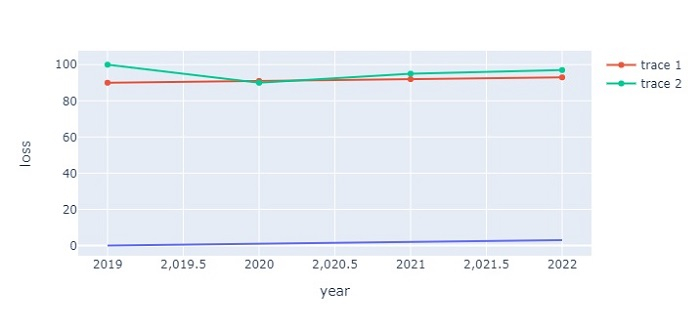# How to draw a multiple line chart using Plotly Express in Python Plotly?

Plotly is an open-source plotting library in Python. Python users can use Plotly to generate different types of interactive web-based charts including scientific charts, 3D graphs, statistical charts, financial charts, etc.

In this tutorial, we will show how you can use Plotly to generate multiple line charts. Here we will use plotly.express to generate figures. It contains a lot of methods to customize the charts and render them into HTML format.

Follow the steps given below to generate a multiple line chart using Plotly Express.

Step 1

Import the plotly.express module and alias as px.

import plotly.express as px


Step 2

Create a dataset with the following values −

data = {
'year':[2019,2020,2021,2022],
'loss':[0,1,2,3],
'gain':[90,91,92,93],
'profit':[100,90,95,97]
}
df = pd.DataFrame(data)


Step 3

Use the px.line() method to create a line plot.

fig = px.line(df, x='year', y='loss')


Step 4

Use the add_scatter() method to generate two scatter plots.

# generate scatter plot


## Example

The complete code to create a multiple line chart is as follows −

import plotly.express as px
import pandas as pd

# Create a dataset
data = {
'year':[2019,2020,2021,2022],
'loss':[0,1,2,3],
'gain':[90,91,92,93],
'profit':[100,90,95,97]
}
df = pd.DataFrame(data)

# generate the line plot
fig = px.line(df, x='year', y='loss')

# generate scatter plot

# Set the size of the plot
fig.update_layout(width=716, height=350)
# show the plot
fig.show()


## Output

It will show the following output on the browser −Updated on: 21-Oct-2022

3K+ Views